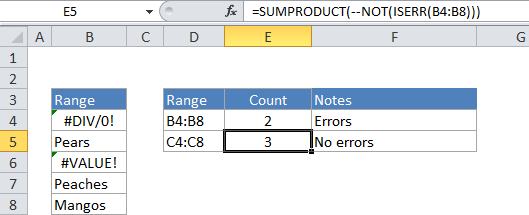## Excel Office

Excel How Tos, Tutorials, Tips & Tricks, Shortcuts

# Count cells that do not contain errors in Excel

This tutorial shows how to Count cells that do not contain errors in Excel using the example below;

## Formula

`=SUMPRODUCT(--NOT(ISERR(range)))`## Explanation

To count the number of cells that contain errors, you can use the ISERR and NOT functions, wrapped in the SUMPRODUCT function.

In the example, the active cell contains this formula:

`=SUMPRODUCT(--NOT(ISERR(B4:B8)))`

### How this formula works

SUMPRODUCT accepts one or more arrays and calculates the sum of products of corresponding numbers. If only one array is supplied, it just sums the items in the array.

Also See:   Check if multiple cells have same value with case sensitive in Excel

The ISERR function is evaluated for each cell in range. Without the NOT function, the result is an array of values equal to TRUE or FALSE:

{TRUE;FALSE;TRUE;FALSE;FALSE}

With the NOT function, the result is:

{FALSE;TRUE;FALSE;TRUE;TRUE}

This corresponds to cells that do not contain errors in the range.

The — operator (called a double unary) forces the TRUE/FALSE values to zeros and 1’s. The resulting array looks like this:

Also See:   How to count specific characters in a range in Excel

{0;1;0;1;1}

SUMPRODUCT then sums the items in this array and returns the total, which in the example is the number 3.

You can also use the SUM function to count errors. The structure of the formula is the same, but it must be entered as an array formula (press Control + Shift + Enter instead of just Enter). Once entered, the formula will look like this:

`{=SUM(--NOT(ISERR(B4:B8)))}`

Don’t enter the braces {}, they are entered for you when you press Control + Shift + Enter.

Also See:   Count cells that contain odd numbers in Excel